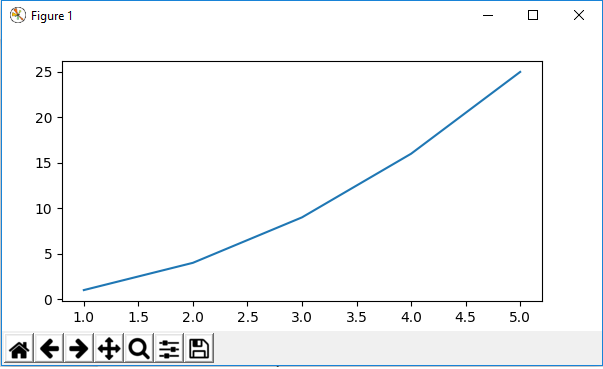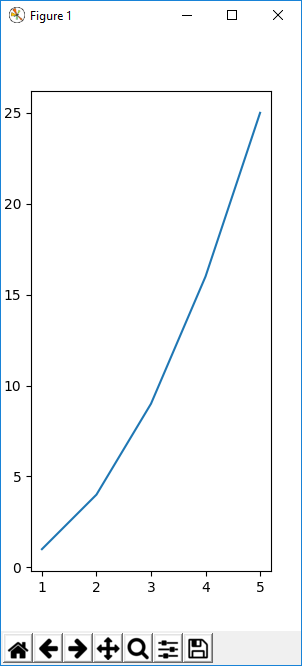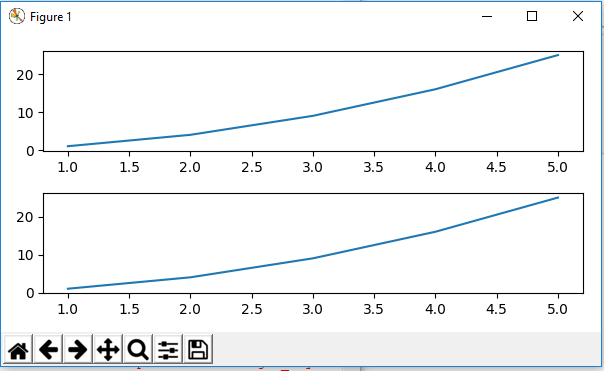﻿ How to Set the Size of a Figure in Matplotlib with Python ﻿# How to Set the Size of a Figure in Matplotlib with PythonIn this article, we show how to set the size of a figure in matplotlib with Python.

So with matplotlib, the heart of it is to create a figure.

On this figure, you can populate it with all different types of data, including axes, a graph plot, a geometric shape, etc.

We may want to set the size of a figure to a certain size. You may want to make the figure wider in size, taller in height, etc.

We can do this with matplotlib using the figsize attribute.

The figsize attribute allows us to specify the width and height of a figure in unit inches.

So let's see how to do this in matplotlib with Python.

So the first thing we have to do is import matplotlib. We do this with the line, import matplotlib.pyplot as plt

We then create a variable fig, and set it equal to, plt.figure(figsize=(6,3))

This creates a figure object, which has a width of 6 inches and 3 inches in height.

The values of the figsize attribute are a tuple of 2 values.

The width is the first parameter of the figsize attribute and the height is the second parameter. So it is, figsize(weight,height)

The width is double the height with this setup.

We then add axes to this figure and then plot a graph for the figure.

To show this figure object, we use the line, fig.show()

This works if you're using a python IDE other than jupyter notebooks. If you are using jupyter notebooks, then you would not use, plt.show(). Instead you would specify in the code right after importing matplotlib, %matplotlib inline

This line allows the figure of a graph to be shown with jupyter notebooks.

After running the following code above, we get the following figure shown in the image below.So now you see a figure object with the size of the width double the size of the height.

Just to show you the dynamic nature of figure sizing in matplotlib, now I'm going to create a figure with the dimensions inverted. The height will now be double the size of the width.

This is shown in the following code below.

So now we have the height double the width.

This produces the following graph shown below.So now you see that the height of the figure is double the width.

You can also set the size of subplots in this same manner.

The following code below sets the size of subplots in matplotlib.And this is how to set the size of a figure in matplotlib with Python.

Related Resources

How to Randomly Select From or Shuffle a List in Python